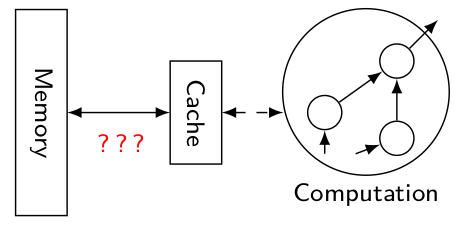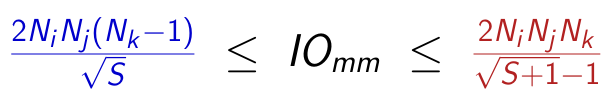IO Complexity lower and upper bounds

is a tool that computes symbolic bounds on the I/O complexity of a program, which is the minimal volume of communication required to perform a computation. It is composed of two parts:

• IOLB which computes a symbolic lower bound
• IOUB which computes a symbolic upper bound

Research article - automatic derivation of a lower bound of IO complexity [PLDI'2020] Research article - automatic derivation of an upper bound of IO complexity [PLDI'2021]

## What is I/O complexity ?

Let us consider a simple memory model composed of:

• a large memory (Memory) which contains initially all the input data of a program
• a small memory (Cache) of size S, such that the data used and produced by a computation must be in this memory.Because of the limited size of the cache, some data might need to be transferred several times between the Cache and the Memory. The I/O complexity is the minimal amount of data needed to be transferred, for any schedule of the computation.

Here is an example of upper and lower bounds which were derived for the matrix multiplication computation. The Nx are the sizes of the matrices, and S is the size of the small memory:## Try it!

Try the IOLB demo Try the IOUB demo

The code is also available on Gitlab: IOLB and IOUB